﻿ Content and Semiotic Features of Mathematical Problems Used as a Means of Training the Primary School Education Students

### Content and Semiotic Features of Mathematical Problems Used as a Means of Training the Primary Schoo...

Nina Tarasenkova, Oksana Kovalenko

American Journal of Educational Research

## Content and Semiotic Features of Mathematical Problems Used as a Means of Training the Primary School Education Students

Nina Tarasenkova1,, Oksana Kovalenko1

1Bohdan Khmelnytsky National University of Cherkasy, Cherkasy, Ukraine

### Abstract

The article focuses on semiotic and semantic features of the mathematical tasks which are set in the course of teaching mathematics to primary school education students. The data are analysed and experimentally confirmed.

• Nina Tarasenkova, Oksana Kovalenko. Content and Semiotic Features of Mathematical Problems Used as a Means of Training the Primary School Education Students. American Journal of Educational Research. Vol. 3, No. 12B, 2015, pp 31-35. http://pubs.sciepub.com/education/3/12B/7
• Tarasenkova, Nina, and Oksana Kovalenko. "Content and Semiotic Features of Mathematical Problems Used as a Means of Training the Primary School Education Students." American Journal of Educational Research 3.12B (2015): 31-35.
• Tarasenkova, N. , & Kovalenko, O. (2015). Content and Semiotic Features of Mathematical Problems Used as a Means of Training the Primary School Education Students. American Journal of Educational Research, 3(12B), 31-35.
• Tarasenkova, Nina, and Oksana Kovalenko. "Content and Semiotic Features of Mathematical Problems Used as a Means of Training the Primary School Education Students." American Journal of Educational Research 3, no. 12B (2015): 31-35.

 Import into BibTeX Import into EndNote Import into RefMan Import into RefWorks

### 1. Introduction

At the present stage of the development of Ukraine there have taken place significant changes in economic, social, and humanitarian sector. There is a growing need for new concepts in educational reforms to update the content of professional training, methods and technologies of educational process. Beginning with primary school, searching for ways and means of creating the most favorable conditions for productive education and development of each student is the main task of modern schooling. In this regard, professional training of future teachers needs updated approaches.

In the school system of primary level a teacher occupies a special place due to the necessity of formatting the basics of analytical, conceptual, operational, linguistic and ideological backgrounds of the elementary school students. Updating approaches to teaching mathematics to the primary school education students is not limited to studying the latest developments of mathematics as a science, the logical organization of subject content, and the use of modern teaching methods, forms and tools. Mathematical training of the primary school education students often initiates internal contradictions : between new cognitive purposes and existing methods of operation, new situations and previous experience, generalizations that have been already established and new facts. The main task of future teachers’ training is a vigorous activity aimed at eliminating such contradictions and establishing a kind of relationship between the course of school mathematics and the course of mathematics at the university.

### 2. Methodology of Research

According to many scientists [1, 2, 3, 10, 13, 14], the main and the most difficult part of both pupils’ and students’ activities in studying mathematics is solving problems.

The problem of teaching how to solve math problems is highlighted in numerous works of Ukrainian and foreign scholars and researchers (I.A. Akulenko, G.A. Ball, M.I. Burda, N.B. Istomina, Y.M. Kolyahin, D. Polya, Z.I. Slyepkan, L.M. Fridman etc.). Particularly, the system of educating problems in teaching mathematics to students was studied by I.M. Bogatyreva; O.V. Barninovoyi, N.V. Bogdanovich, G.P. Lyshenko, V.V. Malykhina, V.A. Mizyuk, S.O. Skvortsova - their works address the issues of teachin the education students to solve mathematical problems; the particular features of interaction between a teacher and a student of the elementary school when dealing with solving problems are highlighted in the works of Y.S. Hayevets and L.V. Dyachenko; the problem of developing the mathematical culture of primary school education students is explored by E.O. Lodatko. All researchers argue that the ultimate goal of their studies is to facilitate students' abilities to solve mathematical problems. A primary school teacher is a key figure in it.

However, the analysis of scientific and methodical literature shows that the problem of teaching mathematics to the primary school education students has not got proper coverage so far. Hence, scientific and methodical work on the issue still goes on.

2.1. Content Features of Mathematic Problems

Solving any problem begins with reading the text of the problem. First, students should understand the conditions - what is given, and the demands of the problem, that is – what they are asked to do, and how they are to represent the results obtained. According to the requirements set in them mathematical problems are divided into four types - problems on the calculation, problems for finding proof, problems on construction, and research problems [4, 5, 7, 11, 12]. The success of the first step in the analysis of the text (decoding the original data) and, in our opinion , the entire course of the next solution depends on the specific semantic-symbolic shell, which wraps the problem. But attributing the problem to a particular type of analysis is an important aspect of its formulation. Solving problems requires a certain type of relevant subject (the use of a particular method or way of solving problems using identical changes, substitution of numerical values into the formula, a method of proof, etc.).

In the school course math problems on calculations constitute the widest class of problems. These are the problems which the pupils are to solve at their first classes of mathematics at school. These tasks include text (narrative) task, and the task in which you are to calculate the value of numerical expression, to substitute the numeric data in a formula, to convert alphabetic or numeric expression, and to solve the equation or the inequality.

If problems ask for establishing mathematical facts (of general or individual character), these are the problems on finding the proof. In one case, the problem is a theorem, in another - the problem for proving a mathematical fact of a particular character.

The class of problems on construction includes geometric problems (with the requirement to build a geometric figure) and arithmetic or algebraic tasks aimed at creating charts, graphs, functions, etc. Students can solve these problems when they have some knowledge and skills in the subject. Research problems set the tasks of investigating certain mathematical laws, determining the conditions of existence of certain mathematical objects, and defining the features of problem solutions .

In the primary school mathematics course the vast majority of problems belong to the first type, i.e. - calculation. Basic problems on construction ("using the ruler construct an acute and obtuse angle", "Draw a circle the radius of which equals ...", "Construct a rectangle with sides ...", "Construct two different rectangles ..." "Draw a segment the lenghth of which would be ..." [4, 5, 6, 7]) and finding the proof (in elementary school such problems are mostly related to proving the accuracy of the performed operatrions, particularly when performing tasks such as "Check the correctness of calculations", "Check the solution" [4, 5, 6, 7, 11, 12]) are less common. In our view, research problems that are offered to the pupils of primary school include problem-questions ("Is it possible to calculate in two ways?", "How to distribute the figures in two groups?", "Signs of which arithmetic actions are missing?" [4, 5, 6, 7, 12]), tasks that require comparison and well-grounded choice of one available option ("Explain different ways of calculating", "Calculate in the most convenient way" [6, 7]), the problem of reconstructing ("Make the problem with a short record" , "Make several problems based on the expression" [4, 5, 6, 7, 11, 12]), and logical problems, such as (№ 416 from : "There are two cans with the capacities of 10 liters and 3 liters. How can one bring 8 liters of water from the river using these two cans? " Such wording encourages pupils/students to find ways of solving, reason logically, rerform creatively, and developm mathematical thinking.

In teaching mathematics to the primary school education students and when choosing the tasks for practical and laboratory classes, independent do-it-at-home performance and monitoring students’ learning it's worth creating a system of learning tools that will contain every type of problem.

2.2. Semiotic Features of Mathematical Problems

As it has been said above , semantic-symbolic shells of the problems are created by means of natural language, logical-mathematical symbols, and other iconic and symbolic means. The tasks formulated by means of the mother tongue are not necessarily the simplest. This characteristic of the texts of math problems is obviously of considerable importance for the procedures of comprehending the initial information, but it gets the appropriate content (and hence, should be analyzed in detail) only in connection with a substantive content of the problems and their didactic purpose.

The most common features of the texts of mathematical problems include:

a) application of drawings, tables, and graphs that alongside with text fragments carry essential information about the data of the problems;

b) including nonlinguistic elements into the text of the problem (as topographically lumped and distributed);

c) formulating the problems in the form of n-ary texts (it concerns math sums).

The features named above have different effects on the process of analyzing the problem. The impact of the first characteristic on the difficulty of the problem can be defined only in connection with its substantive content and didactic purpose, but it only when the second characteristic is available that it is possible to say that the text of the problem is extremely difficult (due to the physiology of the human analytical system). The wording of several problems in the form of n-ary text makes them more compact and easier for comprehending (it is important how the individual fragments are arranged respective of each other and the common text fragment of the problem).

In order to efficiently teach the primary school pupils a teacher should be able not only to solve the problem of some kind, but also to analyze each problem from the point of view of its complexity for the pupils/students (according to two parameters - semantic and semiotic), to build a differentiated system based on two parameters.

### 3. Discussion and Results

In order to implement the propedeutics of the methodical preparation of the primary school teachers in mathematics in general, and teaching students to analyse the formulation of the problems (semantic and semiotic), it's appropriate to begin it when studying the course "Mathematics". This course is a part of a cycle of training the "Primary education" specialty students and is studied in the first semester. However, as it has pointed out earlier , cooperation and interaction of teachers and students, students with each other, independent tasks, joint or independent decisions, responsibility for the consequences - all of these are the important factors of growth, self-affirmation, and self-expression of a future professional, they ensure the cultivation of mathematical competence.

Let’s consider the examples of problems from the course "Mathematics" taught to the primary school education students and focus our attention on semiotic and semantic features of mathematical problems employed in the educational process.

Task 1. Find the unknown element :

а)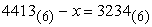; б)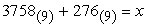;

в)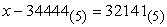; г)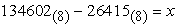.

Task 2. Perform an operation :

а)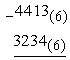; в)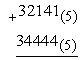;

б)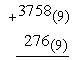; г)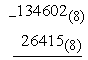.

Task 1 and Task 2 are selected based on different semiotic ways of recording conditions of problems. It is clear that the second design of the n-ary text is easier for decoding its semantics and students better cope with it. Here each numeric expression is topographically separated from the others and it is easier to process it, and secondly, matrix registration of the records which is devoid of odd words explicitly shows similar and different moments in these expressions, and hence gives hints at different approaches to solving them. Thus additional conditions for the improvement of mathematical experience of future teachers are created.

The study involved 28 students who were present at the class. The resulys are given in Table 1.

As can be seen from Table 1, the students coped with the first task worse than with the second one. The first problem, according to students, has a more complicated figuration. The stating of the problem is not explicit enough. Unfortunately, among those primary school education students who participated in this study there are such students who do not differentiate between mathematical operations that are to be petrformed to find an unknown element and mix bit units of the system numbers. The second problem, according to the students, is easier because the expressions are clearly separated from each other and a corresponding text of every mathematical expression explicitely points out the operation which is to be performed. Bit units of the system numbers are correctly arranged.

To enhance the professional orientation of the course of "Mathematics" which is taught to the primary school education students and for the propaedeutics of their methodical preparation, it's advisable to complement the objectives above, for example, with the task to make for primary school pupils two problems with different representations of the text. The problems should be semiotically related to the problems which have been solved but have different contents.

In the mathematics textbooks for elementary school there are problems with initial date the learning of which takes place after a sequential processing of the texts elements. There are also problems the texts of which are to be reworded (processed) for learning the initial data. The latter ones present substantial difficulties for the students in the process of solving. Teachers should do their best to help students understand the content of the problem and get the correct answer. The primary school education students should possess well-developed skills of the prompt identifying what type of problems this or that problem belongs to. At a practical class on "Geometric values. Volume of the geometric body and its measurements" the following two problems were offered to students:

Task 3. The first edge of the rectangular parallelepiped is 16 cm, the second edge is 2 times larger than the first, and the third one is 4 cm smaller than the first. Find the volume of the rectangular parallelepiped.

Task 4. The first edge of the rectangular parallelepiped is 32 cm, it's 4 times larger than the second edge and 11 cm smaller than the third one. Find the volume of the rectangular parallelepiped.

The picking number of the participants of our study is the same - 28 students. The results are given in Table 2.

These tasks are performed by the students singlehanded. Table 2 shows that the wording of the third problem has not caused any problems during the analysis and processing of the text of the task. It has consistently and clearly stated all the components that indicate the performance of the relevant arithmetic operations. Two students who partially completed this task, made a mistake in finding the third edge of the rectangular parallelepiped (in our view, this happened because of their absent-mindedness). The wording of the fourth task somewhat confused the students during the analysis of the conditions and, consequently, the performance of arithmetic operations. Although the problem was not difficult, it caused a need for the reformulation of the text that was done by us after checking the performance of both tasks.

When solving problems for proving mathematical facts of a specific character, students should be motivated to realize that the value of solving such problems lies not so much in the assertion of the facts formulated in the problems but in improving their own possibilities by refining the skills of identifying the initial data, analyzing the formulation of the problem, choosing methods or manners of proving and the means necessary for their application, and, finally, arguing covincingly.

Here are some examples of such problems.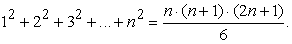In studying the axiomatic construction of the set of nonnegative integers, Peano axioms in particular, the method of mathematical induction is considered. When offering Task 5 to the students we follow the goal to develop the students' skills of differentiating the steps of proving by a certain method of substantiation steps and the ability to make assumptions and draw conclusions about the truth of the statement.

Task 6. Points A (7.5; -1) and B (1.5; 4) are the ends of the segment. Check it whether point C (4.5; 1.5) is the middle of the segment.

After learning the basic material of the theme "Geometric values. The length of the segment and its measurement ", through Task 6 we are to test the ability of the students to seek the middle of a given segment primarily by means of appropriate formulas (formulas of the coordinates of the middle of the segment). However, if students do the task correctly only graphically, the result is to be accepted but with a lower grade.

The results of the performance of the tasks are given in Table 3. The number of respondees is the same - 28 students.

Based on Table 3 it can be affirmed that performing the fifth task is difficult for the majority of the students who participated in the experiment. All but five people confuse those stages of proving that are based on the method of mathematical induction. Four students, unfortunately, have not understand the essence of this method. Task 6, as seen from Table 3, was performed by the majority of the primary school education students. The solving of this problem required the inserting of the coordinates of points A and B in the appropriate formulas and comparing the result with the coordinates of point C. Of the 22 students who completed the task, 18 performed successfully and 4 students did the task only graphically.

While working with the simplest mathematical problems, both – the primary school pupils and the primary school education students should be assimilating instructions as to solving problems and understand that solving mathematical problems helps in self-improvement. The slightest success should be encouraged.

Practice shows that in teaching mathematics there are problems in solving which several ways are possible or the there is an initial requirement to solve the problem in several ways. We call them content-alternative problems  and in teaching younger pupils we refer them to the basic research problems. Such situations are encountwred in solving both algebraic and geometric problems. Employing certain instructional tools and techniques it is possible to effectively teach pupils and students to select several points of entry into the decoding, and this way to teach them solve problems in several ways. Such techniques include, for example, the system of leading questions or tips, and the method "from the least obvious to the most obvious." Of course, if from the very beginning the students are not set on finding several ways of solving the problem, the effectiveness of any further actions objectively can not be high. Barriers in finding several ways of problem solving mostly arise from a number of stereotypes imposed either by the formulation of the problem, or the pressure of the very context of the course topics, or by the teaching methods, or by the lack of interest in the subject.

Consider, for example, the following tasks taken from themes of the Mathematics course studied by the primary school education students, these are the tasks that can be solved at least in two ways.

Task 7. Find the greatest common divisor (GCD) and the least common multiple (LCM) of the numbers 6253 and 1001.

This task, as well as similar tasks thereto, was offered in the frameds of the theme "The divisibility of numbers." It assumes two ways of finding GCD and LCM – through using the Euclidean algorithm and through the decomposition of numbers into prime factors. If the students are instructed that the task can be performed in two ways, the vast majority of them solves it only in one way – through the decomposition of numbers into prime factors. Students find this way easier to remember, and hence - to use, they consider it to be more common, more compact and less time-consuming. A leading question "What ways of finding GCD and LCM of numbers do you know?" helps students understand that this problem has two ways of solving and they are to examine these ways.

а) 479 + 1796 + 1631 + 8204;

б) (72 + 54) : 9;

в) (44 ∙ 23) : 22.

This task is on the theme "Theoretical and multiple contents of natural numbers, a zero, and operations with numbers" which primary school education students study in the course of Mathematics. Mathematics of primary abundons in the tasks of the kind. Typically, both students and pupils complete such tasks paying attention only to the sequence of operations. Only arter they are said about the possibility of solving this problem in different ways, both pupils and students begin to analyze the condition of this problem considering its components (some of them remember a convenient way to perform operations). However, after the tips are given pupils and students easily do in two ways the first sum of the three ones from Task 8, but the doing the second and the third sums causes a considerable difficulty. And it is only a leading questions "What laws of arithmetic operations do you know?" that the majority of the students didn't fail to achieve the results expected by the teacher.

Task 9. Points K, M, and N are collinear. The distance from point C to point M is 6 times larger than the distance from K to N. Find the distance between points K and N, if the distance between M and N is 35 cm.

This problem is on the theme "Geometric values" studied also by both – the primary school pupils and the primary school education students. And for both this task is quite difficult. Solving it requires a corresponding development of mathematical thinking. The use of the method "from the least obvious to the most obvious" this method lies in sorting out all possible cases of placing three points on a straight line (N lies between K and M; K is between M and N; M lies between K and N). Not everyone can solve this problem singlehanded.

The results of tasks 7 - 9 are present in Table 4 (The number of respondees is 28 students).

#### Table 4. Results of performing Tasks 7, 8 і 9

Judging by the results given in Table 4, we can conclude the following: the ability of students to find different ways of solving problems requires considerable improvement. The system of tasks for every theme from the course of Mathematics must contain problems with the alternate content.

### 4. Conclusions

In our opinion, teaching mathematics both to students and pupils should be based on the principle of consistent distancing from the personal experiences. This is especially important in the early stages of studying mathematics. It is didactically advisable that content and form of problems should allow to create the conditions for the independent transfer of knowledge from familiar situations to the unfamiliar ones. Here all the requirements to the differentiated system of problems should be implemented. Knowledge and skills acquired in the process of solving problems in mathematics should act as a means of a personal development of a primary school education student. In searching or selecting different ways of solving the problem, reformulating the initial data and conditions, preparing new mathematical problems, and building algorithms for proving this or that mathematical fact students become more conscious participants in the educational process. That is why after studying the course "Mathematics" the primary school education students should be able to : apply knowledge of theoretical foundations and technologies of the elementary mathematics education; use the methods of imaginative and logical thinking; generate substantive abilities and skills in pupils; foster the interest in mathematics and willingness to use mathematical knowledge in everyday life in the primary school pupils.

### Acknowledgement

The work was supported by the Ministry of Education and Science of Ukraine (state registration number 0115U000639).

### References

  Akulenko, I.A., “Role of practical content problems in the study course “Algebra and Number Theory”, Bulletin of Cherkassy University. Series: Pedagogical scienc, publishing house of Bogdan Khmelnytskyy National University at Cherkasy (Issue No 101), Cherkasy, 136-140, 2007.In article  Bogatyreva, I.M., Methodology of development and introduction of a system of developing problems in teaching mathematics pupils 5-6 forms: thesis, Bohdan Khmelnytsky National University at Cherkasy, Cherkasy, 2009, 227.In article  Bogatyreva, I.M., “About strengthening the function of developing problems in the mathematics of 5-6 forms”, Mathematics in school, No 6, 27-32, 2008.In article  Bogdanovich, M.V., Mathematics: Textbook for 1 class, “Osvita”, Kyiv, 2007, 144.In article  Bogdanovich, M.V., Mathematics: Textbook for 2 class, “Osvita”, Kyiv, 2010, 160/In article  Bogdanovich, M.V., Mathematics: Textbook for 3 class, “Osvita”, Kyiv, 2006, 160.In article  Bogdanovich, M.V., Mathematics: Textbook for 4 class, “Osvita”, Kyiv, 2007, 160.In article  Kovalenko, O.A., “Organization of the work students in small groups”, Materials of XVI Ukrainian with international scientific-practical correspondence conference (26-27 December 2014), “GO IOMP”, Kyiv, 180-182, 2014.In article  Kukhar, V.M., Tadiian, S.I., Tadiian V.P., Mathematics: Sets. Logic. Integer: Practicum, “Vyscha shkola”, Kyiv, 1989, 333.In article  Lodatko, E.O., Theory and practice of mathematical culture of the elementary school teacher: thesis, Slaviansk, 2011, 566.In article  Skvortsova, S.O., Onopriienko, A.V., Mathematics. Grade 1: 1 h., “Ranok”, Kyiv, 2012, 144.In article  Skvortsova, S.O., Onopriienko, A.V., Mathematics. Grade 1: 2 h., “Ranok”, Kyiv, 2012, 144.In article  Skvortsova, S.O., Methodical system of training of primary school pupils scene solving mathematical problems: thesis, Odessa, 2008, 624.In article  Skvortsova, S.O., “Formation in younger students of the overall ability to solve problem”, Proceedings of Berdyansk State Pedagogical University, No 4, BDPU, Berdyansk, 65-71, 2006.In article  Tarasenkova, N.A., Kirman, V.K., “The content and structure of mathematical competence of students of secondary schools,” Mathematics in school, No 6, 3-9, 2008.In article  Tarasenkova, N.A., Kovalenko, O.A., “Methodical features of mathematics teaching primary school teachers”, Pedagogy of higher and secondary schools, Issue 42, “Typography of Roman Kozlov”, Krivoy Rog, 75-80, 2014.In article  Tarasenkova, N.A., Kovalenko, O.A., “Peculiarities of training course "Mathematics" primary school teachers”, Bulletin of Cherkassy University. Series: Pedagogical science, Issue 36 (249), 114-120, 2012.In article  Tarasenkova, N.A., The theoretic-methodical principles of using of the sign and symbolic means in teaching mathematics of the basic school students: thesis, Bohdan Khmelnytsky National University at Cherkasy, Cherkasy, 2003, 630.In article